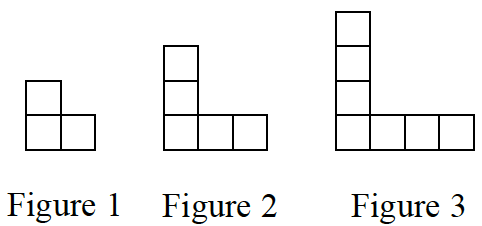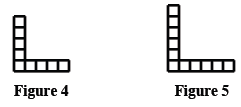Home > CCAA8 > Chapter cca1 > Lesson cca1.1.1 > Problem1-6

1-6.

Examine the tile pattern below. 1-6 HW eTool (CPM)  Homework Help ✎1. On your paper, sketch Figures 4 and 5.

To sketch the next figures in the pattern, you must find out what the pattern is.

How do the figures change as the pattern continues?2. How does the pattern grow? Explain how you know.

See the hints for part (a).

The pattern grows each time by two tiles, one on each side of the figure.

3. How many tiles will there be in Figure 0 (the figure before Figure 1)? Explain how you know.

Once you find the answer, remember to explain how you got to that number of tiles. Also, see part (b).

Use the eTool below to sketch figures 4 and 5.
Click the link to the right for a full version of the eTool: CCA 1-6 HW eTool